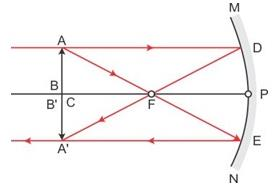Courses

# Test: Geometric Optics (Competition Level 1)

## 30 Questions MCQ Test Physics Class 12 | Test: Geometric Optics (Competition Level 1)

Description
This mock test of Test: Geometric Optics (Competition Level 1) for Class 12 helps you for every Class 12 entrance exam. This contains 30 Multiple Choice Questions for Class 12 Test: Geometric Optics (Competition Level 1) (mcq) to study with solutions a complete question bank. The solved questions answers in this Test: Geometric Optics (Competition Level 1) quiz give you a good mix of easy questions and tough questions. Class 12 students definitely take this Test: Geometric Optics (Competition Level 1) exercise for a better result in the exam. You can find other Test: Geometric Optics (Competition Level 1) extra questions, long questions & short questions for Class 12 on EduRev as well by searching above.
QUESTION: 1

### A point source of light is placed in front of a plane mirror.

Solution:

Plane mirror always forms a virtual image of the real object for all the rays emitted by the object. So, when a point source of light is placed in front of a  plane mirror, all the reflected rays meet at a point when produced backward.
Option B is correct.

QUESTION: 2

### A point object is kept in front of a plane mirror. The plane mirror is doing SHM of amplitude 2cm. The plane mirror moves along the x-axis and x-axis is normal to the mirror. The amplitude of the mirror is such that the object is always infront of the mirror. The amplitude of SHM of the image is

Solution:

Assume the mirror is at the origin and it is moving about the origin along x direction between x = -2 and x = +2 as its extreme positions.
Assume that the object is placed at co=ordinate of x = - (2+x)
So, when mirror is at position x = -2, the object's image will be formed at x coordinate X = - 2+x
And when the mirror is at position x = +2, the image is formed at X = 6+x
So, the net difference in the position of image between these 2 co-ordinates is ( 6 + x  ) - ( -2 + x ) = 8
So, the amplitude will be half of this distance. So the answer is 8/2 = 4 cm.
Option C is correct.

QUESTION: 3

### A watch shows the time as 3 : 25. What will be the time that appears when seen through a plane mirror ?

Solution:

The relation between actual time and given time is:
Actual time=11:60 −given time
Actual time=11:60−3:25=8:35

QUESTION: 4

If a ray of light is incident on a plane mirror at an angle 60° from the mirror surface, then deviation produced by mirror is

Solution:

According to the law of reflection, the angle of incidence and angle of reflection are equal. Both these angles are measured with respect to a line normal to the reflection surface. If I understand the question correctly, the incident ray makes an angle of 60 degrees with the mirror surface, then the angle of incidence can be calculated as:

Angle of incidence = 90 - angle made with the mirror surface

= 90 - 60 = 30 degrees.

Since, angle of incidence = angle of reflection = 30 degrees.

The incident ray will have an angle of reflection of 30 degrees (made with a surface normal to the mirror surface).

The reflected ray will make an angle of 60 degrees (90 - 30 degrees) with the mirror surface.

QUESTION: 5

When light is reflected from a mirror a change occurs in its

Solution:

When a light wave is reflected from an object , it changes not only it's amplitude but also its phase according to the properties of the object at a particular point.
Therefore, option A is the right answer.

QUESTION: 6

The images of clouds and trees in water always less bright than in reality

Solution:

The images of clouds and trees in water are always less bright than in reality because only a portion of the incident light is reflected and quite a large portion goes mid water.

QUESTION: 7

A rays is incident at an angle 38º on a mirror. The angle between normal and reflected ray is

Solution:

As per the question, rays are falling on the mirror, which cleared that Regular reflection is taking place.
So, As per the laws of regular reflection.
=> ∠incident= ∠reflection
∠incident= ∠reflection=38Ao
Also we know that normal is at 90°to the mirror.
Now, to get the required angle we need to reduce angle of reflection from normal.
=>90Ao-38Ao=52Ao
so, the correct answer is option B.

QUESTION: 8

Mark the correct options

Solution:

If the final rays are converging, we have a real image.
This is because a real image is formed by converging reflected/refracted rays from a mirror/lens.
The correct answer is option B.

QUESTION: 9

A point source of light is placed in front of a plane mirror.

Solution:

Plane mirror always forms a virtual image of the real object for all the rays emitted by the object. So, when a point source of light is placed in front of a  plane mirror, all the reflected rays meet at a point when produced backward.
Option A is correct.

QUESTION: 10

Which of the following letters do not surface lateral inversion

Solution:

Lateral inversion-The phenomenon of left side appearing right side and right side appearing left side on reflection in a plane mirror is called lateral inversion.
Letters that do not show lateral inversion are,
A, H, I, M, O, T, U, V, W, X, Y
Hence, HOX will not show lateral inversion.

QUESTION: 11

An object is initially at a distance of 100 cm from a plane mirror. If the mirror approaches the object at a speed of 5 cm/s. Then after 6 s the distance between the object and its image will be

Solution:

Given that,
The distance of object from plane mirror D=100cm
So, initial distance of image and object
=100−(−100)
=200cm
Now, object approaches the mirror with the speed=v=5cm/s
So, distance travelled by object in 6 sec
D=5×6
D=30cm
At this instant,
Distance between mirror and object
D=100−30
D=70cm
Now, the distance between image and object is
D=70−(−70)
D=140cm
Hence, the distance between the object and its image is 140 cm.

QUESTION: 12

Two mirrors are placed perpendicular to each other. A ray strikes the first mirror and after reflection from the first mirror it falls on the second mirror. The ray after reflection from second mirror will emerge

Solution:

Let ray strikes the vertical mirror by angle of incidence θ. Then by joining the perpendiculars of two mirrors it is clear from the diagram that angle of incidence at the horizontal mirror is (90−θ).

∴ reflected ray from the horizontal mirror makes angle θ with the horizontal mirror also incident ray at vertical mirror makes angle θ with horizontal axis.
∴ reflected ray is parallel to the original ray.
This is true for two perpendicular mirrors fixed at any positions.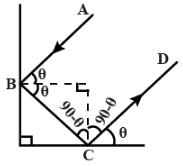QUESTION: 13

A person is in a room whose ceiling and two adjacent walls are mirrors. How many images are formed?

Solution:

The number of images formed when two mirrors are inclined to each other is given by :
n=(360/θ -1)
here θ= 90°[since walls are perpendicular]
so, number of images=n=360/90-1
=4-1
=3
These 3 images are formed by a combination of two adjacent walls with the object itself acts as objects for the ceiling mirror. So totally 4 images are formed.
Hence total number of images formed are 4+3= 7

QUESTION: 14

If an object is placed unsymmetrically between two plane mirrors, inclined at the angle of 600, then the total number of images formed is

Solution:

The number of images between two plane mirror inclined at an angle θ when the object is placed symmetrically between the mirrors is given as
n=(360o​/ θ) −1
Given,
θ=60o
∴n=(360o​/60)−1=6−1=5

QUESTION: 15

In image formation from spherical mirrors, only paraxial rays are considered because they

Solution:

Paraxial rays are ones which originate from a point source and make a small angle with the principal axis. After reflection, they give a point image, either real or virtual.
But if rays from point source that are far from the mirror axis, gives a blurred image, an effect called spherical aberration.

QUESTION: 16

A concave mirror of radius of curvature 20 cm forms image of the sun. The diameter of the sun subtends an angle 1° on the earth. Then the diameter of the image is (in cm)

Solution:

Given: A concave mirror of radius of curvature 20cm forms image of the sun. The diameter of the sun subtends an angle 1o on the earth.

To find the diameter of the image

Solution:

As sun is more far from the earth,

Then we take u = infinite

Radius of curvature, R=−20cm

Focal length,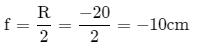From the mirror formula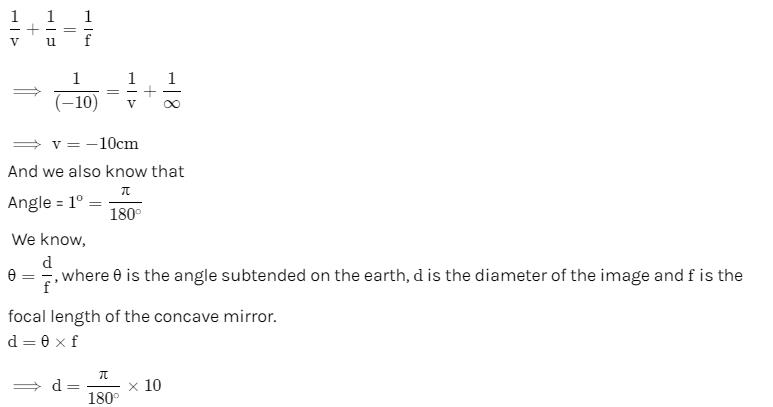d = π/18

QUESTION: 17

A convex mirror has a focal length f. A real object, placed at a distance f in front of it from the pole, produces an image at

Solution:

Here F=f
U=-f(-because it is present in front of a convex lens)
It is given that both focal length and object distance is f .
So according to lens formula 1/F=1/v+1/u
So 1/f=(1/v)+(-1/f)
=>1/f=(1/v)-(1/f)
So (1/f)+(1/f)=1/f
=>1+(1/f)=(1/v)
=>2/f=1/v
So from here we can say that v= f/2
Hence option B is correct.

QUESTION: 18

A convex mirror has a focal length = 20 cm. A convergent beam tending to converge to a point 20 cm behind convex mirror on principal axis falls on it. The image if formed at

Solution:

The converging ray appears to converge on the focus of the convex mirror. Therefore after reflection they will travel parallel to the principal axis. So the image will be formed at infinity.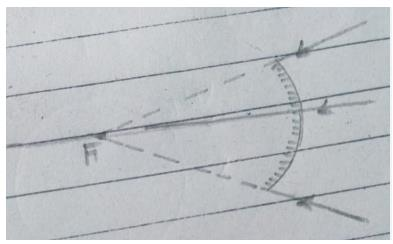Hence,
option (A) is the correct answer.

QUESTION: 19

An object is placed at a distance u from a concave mirror and its real image is received on a screen placed at a distance of v from the mirror. If f is the focal length of the mirror, then the graph between 1/v versus 1/u is

Solution:

For real object and real Image,
(1/v)+(1/u)=(1/f)=> -(1/v)-(1/u)=-(1/f)
(1/v)+(1/u)=(1/f) => 1/v=-(1/u)+(1/f)
y=mx+c
The correct answer is option B.

QUESTION: 20

An infinitely long rod lies along the axis of a concave mirror of focal length f. The near end of the rod is at a distance u > f from the mirror. Its image will have a length

Solution:

At infinity, image of B will form at focus since it is at infinity. Image of A will be at A which can be calculated by mirror formula.
1/v​+(1/−u)​=(1/−f)
⟹v= fu /(f−u)​=−( fu/(u−f)​)
Image length = v−f=(fu/(u−f)​)−(f)

= f2​/(u−f)
(We take the absolute values of the distances to calculate the rod length)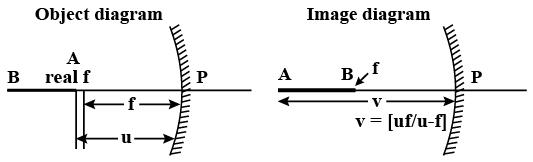QUESTION: 21

The radius of curvature for a convex lens is 40 cm, for each surface. Its refractive index is 1.5. The focal length will be

Solution:

Let the radius of curvature of the convex lens are denoted as
For a convex lens
R1=+40cm [As it is towards right]
R2=-40cm [As it is towards left]
The refractive index of the lens [µ]=1.5
Let f is the focal length of the lens.
From lens maker's formula we know that –
1/f=[µ-1] [1/R1-1/R2]
1/f=[1.5-1][1/40-(1/-40)]
1/f=0.5 x (2/40)
1/f=1/40
f=40cm
Hence, the focal length of the lens is 40 cm.

QUESTION: 22

A concave mirror gives an image three times as large as the object placed at a distance of 20 cm from it. For the image to be real, the focal length should be

Solution:

Given, u=−20cm
m=−3⇒−v/u ​=−3
v=−60cm
1/f=(1/v​)+(1/u)​=(-1/60)​−(1/20)​=−1−3/60​
f=−60/4​=−15cm

QUESTION: 23

If an object is 30 cm away from a concave mirror of focal length 15 cm, the image will be

Solution:

The answer is of the same size because as the focal length of the concave mirror is 15cm then the radius of curvature becomes 30 cm and the object will be on center of curvature by which image will form the same on center of curvature which is inverted and of same length.

QUESTION: 24

A concave mirror cannot form

Solution:

-Virtual image cannot be formed from a virtual object.
- When an object is placed between pole and focus, the image formed by the concave mirror is magnified, virtual and erect.
-When an object is placed beyond the centre of curvature , the image of it is formed between the centre of curvature and focus which is diminished,real and inverted,so the real image of the real object can be formed by a concave mirror.
-When the object is virtual, the image formed is real for the concave surface as shown in figure.

QUESTION: 25

The largest distance of the image of a real object from a convex mirror of focal length 20 cm can be

Solution:

We are given that:
Focal length = 20 cm
In a convex mirror image is formed between focal length and mirror.
So the maximum distance is f i.e. focal length of the mirror.
Now
f = 20 / 2 = 10 cm
Thus the largest distance of an image from a convex mirror is 10 cm.

QUESTION: 26

A particle is moving towards a fixed spherical mirror. The image :

Solution:

1/v + 1/u = 1/f
⇒(-1/v2)x(dv/dt)-(1/u2)(du/dt)=0
dv/dt=-(v2/u2)(du/dt)
For spherical mirror: u is negative and particle is moving towards mirror i.e. u is increasing and du/dt is positive, therefore dv/dt must be negative or v should decrease.
Case-1 ⇒ Image real ⇒ v negative ⇒ decreasing v means image moving away from mirror
Case-2 ⇒ Image virtual ⇒ v positive ⇒ decreasing v means image moving towards mirror
So, image may move towards the mirror

QUESTION: 27

A straight line joining the object point and image point is always perpendicular to the mirror

Solution:

Any line joining the object point and the image point always passes through the centre of curvature of the mirror. This is because of the law, that any ray passing through the centre of curvature of the mirror will retrace its path back.
Thus, any ray passing through the centre of curvature will be perpendicular to the mirror irrespective of the type of the mirror used.

QUESTION: 28

The focal length of spherical mirror is

Solution:

Focal length is the property of a spherical mirror. It does not depend on the light used. So, it is the same for all lights.

QUESTION: 29

A virtual image, larger than the object can be produced by

Solution:

Concave mirror produces a virtual image larger than the object when the object is placed  between the centre of curvature and the pole of the mirror.
Convex lens can produce a virtual and enlarged image when the object is placed between the pole and focus of the lens.

QUESTION: 30

In case of concave mirror, the minimum distance between a real object and its real image is :

Solution:

When an object is placed at the centre of curvature then image is formed at radius of curvature.
So, the minimum distance is zero.
OR
Let focal length be f and an object is placed at u=2f
1/−f=(1/v)+(1/u)=(1/v)+(1/−2f)
v=−2f.
Image will form at the object location.
So the minimum distance is zero.